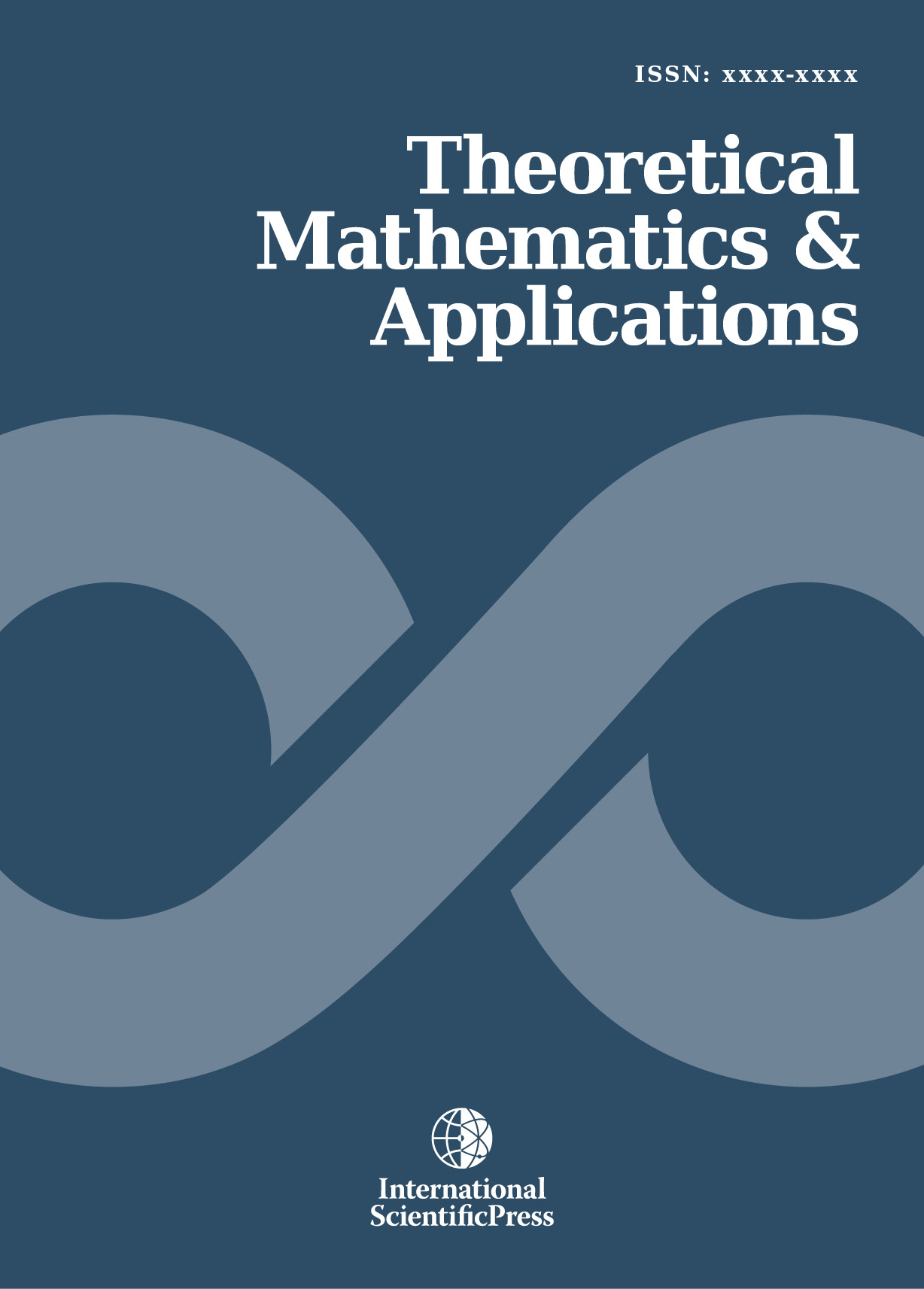# Theoretical Mathematics & Applications

#### Differential equations of amplitudes and frequencies of equally-amplitudinal oscillations of the second order

•[ Download ]
• Times downloaded: 1432
• Abstract

Study of oscillations leads to a need to determine Sturm's two-amplitudinal but equally-frequent equations with positive coefficient (according to results from Prodi ). This is shown by Floké theorem  and monograph Babakov . The problem of finding of amplitudes and frequencies hasn't been solved even in Sturm theory in Amrein et al. , because it is nonlinear problem even though originates from linear oscillations of the second order. In this paper are set up differential equations for amplitude and frequency function of solution of equally-amplitudinal oscillations, and are given theorems on solution existence as well as the important examples.ISSN: 1792-9687 (Print)
1792-9709 (Online)